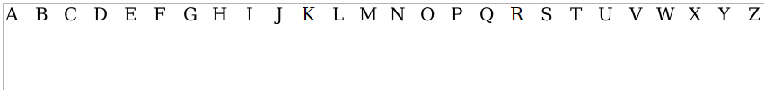Kinematics Quantities - Maple Help

Home : Support : Online Help : Math Apps : Natural Sciences : Physics : Kinematics Quantities

Position, Velocity, Acceleration, Jerk

 Main Concept The motion of objects in the natural world can be described in terms of a number of basic concepts, including position, velocity, acceleration and jerk. The study of these quantities is known as kinematics.   The position of an object, roughly speaking, is a description of how to locate it. Within a given reference frame, an object's position can be described by a displacement vector to the object from the origin of the reference frame. The velocity of an object is the rate of change of this displacement with respect to time. The velocity measures how fast an object moves and in which direction, relative to the origin of the reference frame. In an inertial reference frame, objects move with constant velocity unless acted on by a force, so the velocity can be used to predict where the object will go at a future point in time. The acceleration of an object is the rate of change of its velocity with respect to time. An object experiences acceleration proportional to the sum of all forces acting on it, with the constant of proportionality being the mass of the object. The jerk of an object is the rate of change of its acceleration with respect to time. A jerk occurs naturally when the force an object experiences is changing. Some examples are when collisions occur, or when two magnets are pried apart.   By simulating an object's motion in a one-dimensional world, we can investigate the relationships between these four quantities.

Change the position of the object with the slider. Use the check boxes to specify which graphs you want to observe.More MathApps